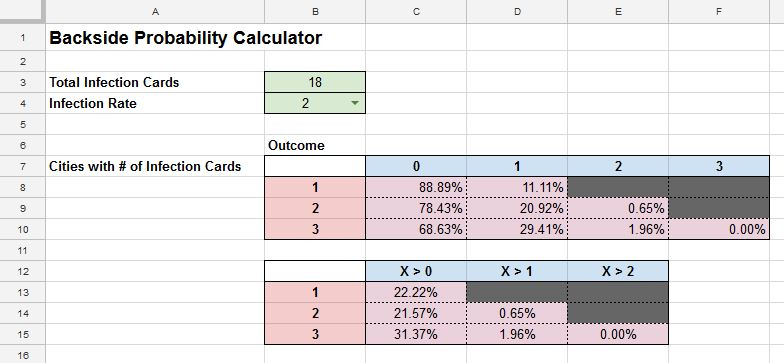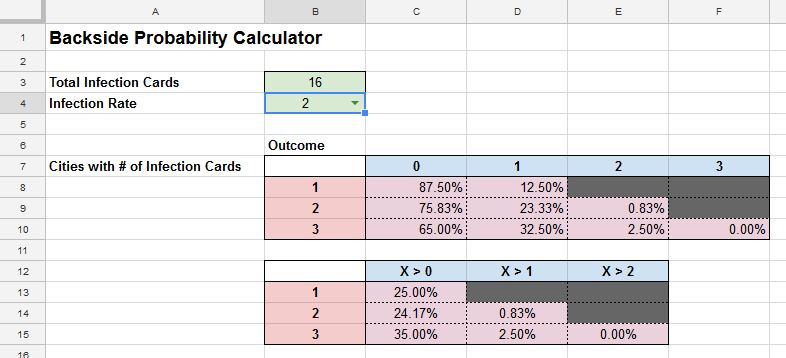# Understanding Probability in Pandemic Legacy: Season 2 – Part 3

Understanding Probability in Pandemic Legacy: Season 2 is a multi-part series. Links to the other parts can be found here:

Understanding Probability in Pandemic Legacy: Season 2 – Part 1
Probability of Incidents During Set-Up

Understanding Probability in Pandemic Legacy: Season 2 – Part 2
Probability of Incidents During Set-Up and How-To Use the Front 9 Calculator

Understanding Probability in Pandemic Legacy: Season 2 – Part 3
Strategies to Survive Between Set-Up and First Epidemic

Strategies to Survive Between Set-Up and First Epidemic

After the infection stage of set-up, what is the best strategy to hold the disease at bay between Set-Up and the first Epidemic?

Why only manage until the first Epidemic? Once the first Epidemic occurs, the discard is shuffled and put back at the top of the deck. Once this happens, we’ll generally have the same subset of cards that we’ll be drawing from after each Epidemic. Right after set-up, we are still drawing from the larger unknown Infection Deck. All we can really do is cover all the cities. Once the Epidemic happens, we’ll know the composition of the next 20 or so cards which can help us determine how many cubes, or none at all are needed on each city and the likelihood that a specific city may be drawn.

Since we already know the contents (deck size and composition) of the Infection Deck, what we do between Set-Up and the First Epidemic is largely based on what cards are revealed during Set-Up.

We can use adapt the Front 9 Calculator to better demonstrate the probabilities during the Infection step at the end of each player’s turns.

The Previous Strategy

The strategy will be similar to that of how we distributed the the supply cubes prior to set-up. We want to minimize incidents by addressing the most vulnerable cities. In the case of distribution of set-up, we distributed the cubes like this:

1. Place one supply cube in each city that has 3 cards in the infection deck.
2. Place one supply cube in each city that has 2 cards in the infection deck.
3. Place one supply cube in each city that has 1 card in the infection deck.
4. Place a second supply cube in each city that has 3 cards in the infection deck.
5. Place a second supply cube in each city that has 2 cards in the infection deck.
6. Place a third supply cube in each city that has 3 cards in the infection deck.

We know that regardless of the deck size, the order (of probabilities) of items #1-6 will always stay true, though as the deck starts to thin, the percentages of an event happening increases.

Deck size = N (Remaining number of cards in the Infection Deck)
Total number of cards drawn = R = 2 (Infection Rate prior to Epidemic 1)
Number of New York cards = n = 3
Number of New York Cards drawn = r = [0, 1, or 2]; r can not be 3 as the most cards that can be drawn is 2.

P(X = r) =

C(n, r) * C(N-n, R-r)
——————
C(N, R)

In layman’s terms,

C(n, r) is the number of ways to draw r New York card from n New York cards.

C(N, R) is the number of ways to draw R (R=2) cards, from N (N = # of cards remaining in the Infection Deck).

C(N-n, R-r) refers to the number of ways to draw R-r (Non-New York) from N-n (Non-New York)

The formula is exactly the same as the Front 9. The biggest difference is the N and R values. Here is the adapted calculator.N=18 (Remaining Deck Size, Cell B3); R=2 (Infection Rate; Cell B4)

A simple substitution of a larger or smaller number in to the Backside Calculator will confirm that the order/priority of the guidelines are still true.N=16 (Remaining Deck Size, Cell B3); R=2 (Infection Rate, Cell B4)

After the 9 infection cards are revealed during set-up, we can use this information to work backwards to determine what cards are remaining in the Infection Deck. The Infection Deck discard is known information. You may look at its content at any time.

Since the order of probabilities is the same, you won’t need to use the calculator. You only need to base your decision from the remaining Infection Deck subset.

Example 1: If two New York cards are revealed during set-up, then there is one New York card remaining in the Infection Deck. Looking at the N=18 chart, the probability that a New York card will be drawn in the next Infection step is 0.1111. This can be found by looking at Row 8 for 1 remaining card in the Infection Deck, and column D, E, and F. The key cell we are looking at is D8 as E8 and F8 are impossible outcomes. (You can’t draw 2 or more cards when there is a fewer number left in the deck.

Example 2: If no New York cards were revealed during set-up. How many New York cards are left in the Infection Deck at the beginning of the game? What’s the probability of this card coming up during the next Infect step (given there is no Epidemic)?

There are three New York cards left in the Infection Deck. Looking at Row 10, and column D, E, F. (Cells D10, E10, and F10.) F10 isn’t a possible outcome and is 0. The sum of D10 and E10 is the probability. Probability = 0.3137.

Revised Guidelines for Game-play Between Set-Up and Epidemic 1

Here’s the revised guidelines. These guidelines are based on the strategy of keeping the most venerable cities stocked with at least one supply cubes. When all cities have at least one supply cube, then does the city receiving a second supply cube is feasible. According to the table provided by the Backside Calculator, it will be very rare to see back-to-back city cards to come out during the Infection step between set-up and the first Epidemic.

1. If a city has 3 Infection cards remaining in the Infection Deck, and there are no cubes on that city, place 1 supply cube on that city.
2. If a city has 2 Infection cards remaining in the Infection Deck, and there are no cubes on that city, place 1 supply cube on that city.
3. If a city has 1 Infection cards remaining in the Infection Deck, and there are no cubes on that city, place 1 supply cube on that city.
4. If a city has 3 Infection cards remaining in the Infection Deck, and there is 1 cubes on that city, place 1 supply cube on that city.
5. If a city has 2 Infection cards remaining in the Infection Deck, and there is 1 cubes on that city, place 1 supply cube on that city.
6. If a city has 3 Infection cards remaining in the Infection Deck, and there is 2 cubes on that city, place 1 supply cube on that city.